# We Helped With This MATLAB Programming Assignment: Have A Similar One?

SOLVEDCategory Programming MATLAB College Solved Do My Signal Processing Homework

## Assignment Description

Assignment instructions:

Please use “main reference file” for reference. The rest is supplement reference. Thanks.

## Assignment Description

Bio 201– Human Physiology 1

Recitation - Cardiovascular Physiology -WindKessel Simulations

Physiological simulations

Rationale underlying simulations of “continuous” systems

Simulations (= mathematical and/or computer models) of physiological systems are used primarily for three reasons:

1)  Explorations of systems that have been simplified (via simplifying assumptions) to concentrate oncertain parts of the system without the complication of noise, biological variation, and the vagaries of  “poorly understood component systems.”  Sometimes the simplification can be so profound that we either do not know whether the system can describe the behavior of the real biological system or even suspect that the model no longer describes the real system.  These simulations are usually undertaken so that we can understand the function of some part of the system under idealized conditions in the hope that the results will help us understand the behavior (or the limits on the behavior) of the real systems with all its warts and wrinkles.

2)  Predictions about the likely behavior of complicated systems under real life conditions.  Thesesimulations (models) must be substantially more complex than the simulations above.  The major problems with such models are that 1) they often strain our ability to describe the system thoroughly enough to describe the behaviors with precision, 2) the level of detail necessary can strain our computational and analytic capabilities.

3)  Simulations with intermediate levels of complexity are often undertaken as our knowledge of asystems develops so that we can explore some new facet of the biology in a more-or-less realistic setting, but still ignoring some of the complications either because they cloud the picture (in this case, the complications can be added to the model after the pattern without them becomes clear) or because they are not well enough understood to add to the model.

In physiology, all three types of models are useful in turning a qualitative understanding of a system into a quantitative understanding (e.g., pressure gradients drive blood flows  - - > how widely will arterial blood flow rates vary through time as the arterial pressure rises and falls  - - > how is oxygen supply to the tissues affected?;    vasa recta appear to be important to the maintenance of the renal medullary concentration gradients - - > how will the gradients be affected if blood flow in the vasa recta is cut by 20% - - > how will that affect the ability to excrete concentrated urine).  Such insights are certainly important in applied physiology (e.g., in medicine), but they can also be important in getting a more basic understanding of the physiology itself.  For instance, we have long thought that the vasa recta must be important to excreting a concentrated urine because they are in a position to affect the renal concentration gradients responsible for a concentrated urine and changes in blood flow rates are associated with different final urine concentrations.  But we depend on models of the renal medulla to help us understand how and why those effects come about.  One of ‘Akin’s Laws of Spacecraft Design’ is that “engineering is done with numbers, all else is opinion.”  In many areas of physiology, the same is true.

Euler simulations stepping rate laws through time Frequently in physiology, the governing relationships for models come in terms of a “rate law” which specifies some ‘rate’ of interest in terms of one or more conditions in the system at the time.  For example, diffusion across a membrane is usually said to be governed by Fick’s Law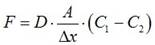(1)

 where F = diffusive flow D = diffusivity A = area Dx = membrane thickness Ci = concentration on either side of membrane

which specifies the rate of diffusion in terms of several parameters (diffusivity, area, membrane thickness) and some “state variables” (C1 and C2).  (Parameters are fixed (or nearly so) and variables change during the simulation.)

Likewise blood and airflow in the cardiovascular and respiratory systems, chemical reactions,  transport rates controlling urinary concentration, and the changes in conductances and voltages in the HodgkinHuxley model, etc) are all described by rate laws.  Usually, the variable of interest (a state variable) is the result accumulation or depletion of the quantity in the rate law.  For instance, in Fick’s law above, the rate (F) gives a flow of molecules across a membrane and the state variables (C1 and C2) are the resultant concentrations of the molecules.

This class of models is an important one in physiology and the approaches to simulating the behavior of the system are, at their base, very simple.  The baseline approach is called an Euler method simulation and is based on the Taylor series that you learned in calculus.  If u(t) is a function (series of values through time) that describes what we want to know (e.g., the membrane voltage, blood pressure, PO2, or [Na+]), and if we know the value at some ‘initial’ time (to), then to get the value of u(t0+Dt) at some time Dt later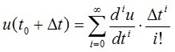(2)

or, in more detail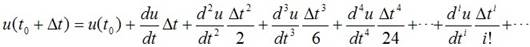(3)

This is great, but all those derivatives can be hard to calculate.  So what happens is that folks ‘truncate’ the Taylor series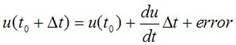(4)

where the error term is the sum of all the terms with ‘higher order’ derivatives and is not estimated.  The idea is to make the error term much smaller than the term with the first derivative, by making Dt (the time step) very small (why would this work ???  - look at Eqs. 3 & 4, then think about what the short time step means physically).

This leads to an algorithm for simulating systems described by rate laws called the Euler method.

1)  Start at an ‘initial’ time for which you know the value of ‘u(to)’.

2)  Use the rate law to get du/dt at this initial time.

Examples: for diffusion - Fick's Law, for blood flow - the "Ohmic" flow equation given in Eq. 5 below.

3)  Using a short time step, estimate the value of u(to+Dt) from Eq. 4.

4)  Treat this value as a new ‘known’ value of ‘u’ at time to+Dt.

5)  Repeat steps 2-4 for this new ‘known’ value to predict a value for u(to+2Dt).  Continue taking short time steps until you have reached the endpoint of the simulation.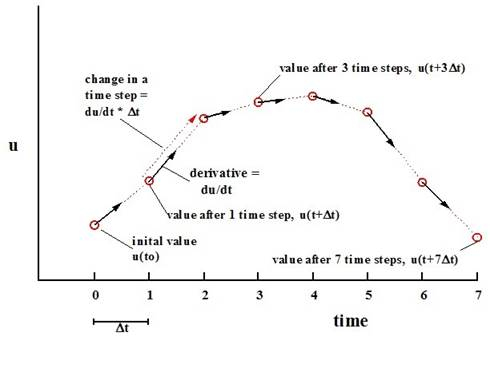### Figure 1.  Summary of the Euler algorithm

Thus one walks through time continually predicting the value of ‘u’ after the next time step, then treating that new predicted value as if it were ‘known’ and using it to predict the new derivative from the rate law. In the WindKessel simulations below, one starts with ‘known’ initial values of volume of blood in the artery (and the blood pressure that said volume causes), the pressure in the heart, and the resistances to blood flow, calculates the blood flows into and out of the artery from those values (Eq. 7a & b), then calculates the rate of accumulation or loss of volume in the artery from the blood flows (Eq. 7c), and the changes in blood pressure that result (Eq. 8).  From these values one can now calculate the blood flows at the next time step, and the new derivatives of the volume in the artery ...     Eventually, one traces out the predicted trajectories for the volumes, flows, and pressures.

There are a number of refinements that are meant to counter some of the weaknesses of the Euler method (mostly associated with the error term that the Euler method assumes is small enough), but they needn’t concern us here.

#### WindKessel effects

Consider a ventricle that pumps blood intermittently into an aorta and arterial tree.  For simplicity’s sake assume for the moment that the ventricle is a piston pump, i.e., one in which a piston pushes on the blood to create high pressures and force the blood along.  Think of the pump as pushing the blood into an aorta that was very stiff (i.e., increased pressure did not stretch the aortic walls very much), rather like a lead pipe (Fig. 2).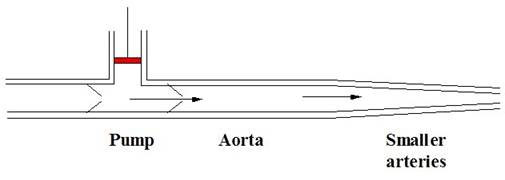Figure 2.  Piston ventricle pumping blood to a stiff aorta.

As the piston pushes on the blood, the pressure in the pump increases.  Because water, the major component of blood, is nearly incompressible (increased pressure does not compress the blood into a smaller volume, increasing the pressure by 760 mmHg would compress water by only about 45 parts per million), as the pressure increases in the ventricle, the blood volume does not change and the major effect is for blood to flow across the valve into the aorta.  As blood enters the aorta the pressure in the aorta rises. Because the stiff aorta cannot expand and the blood cannot be compressed, no blood can be “stored” in the aorta and the amount of blood that flows out of the aorta and into the distal arterial tree must nearly equal the amount that flows in from the ventricle.  (This assumes that the pressure in the aorta doesn’t go sky high and start compressing the nearly incompressible blood, but 1) blood flow will slow and eventually stop as the aortic pressure approaches ventricular pressures, and 2) real ventricular pressures (or those in machinery or at the bottom of the sea) are no way near high enough to significantly compress water.)  As the piston starts its return stroke and stops pushing the blood, the pressure in the ventricle falls and flow into the aorta ceases.  A second consequence of the incompressibility of water and the stiffness of our hypothetical aorta is that as inflow to the stiff aorta ceases, aortic pressure rapidly falls to the ventricular pressure and downstream flow from the aorta to the distal vessels nearly ceases.  With a sufficiently stiff vascular system (think in terms of metal pipes), arterial pressures and flow rates would fall nearly to zero during diastole (Fig. 3).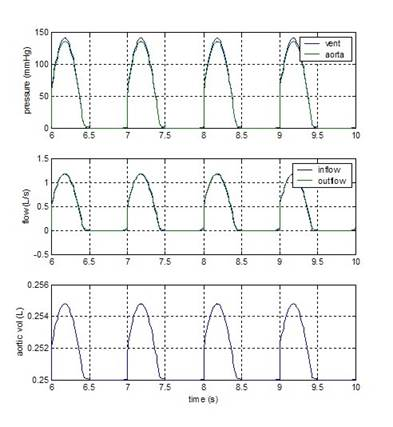Figure 3 Pressures, flows, and volumes with a stiff aorta

Now consider an aorta that is highly distensible like a water balloon rather than stiff like a pipe (Fig. 4).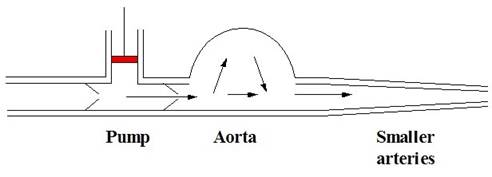Figure 4 Piston pumping to a distensible aorta

In this case as the pressure in the ventricle increases and blood flows into the aorta, some of that blood is “stored” in the aorta because the increased pressure distends the aorta (Fig. 4).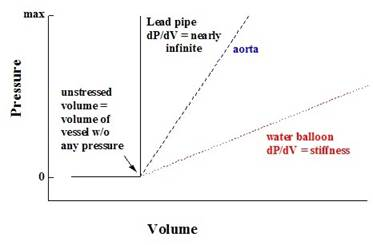Figure 5 Pressure volume relationships in a blood vessel

As a consequence, during systole, inflow to the aorta will exceed outflow to the distal vessels and the aorta will distend and accumulate blood.  At the beginning of diastole, however, when ventricular pressures fall, the blood accumulated by the aorta is still there, so the pressure in the aorta remains elevated and this drives a diastolic flow from the aorta to the distal vessels, draining the blood stored in the aorta and allowing the arterial pressure to fall as the blood flows off distally.  This can affect the mean, maximum, and minimum pressures in the aorta, the timing and the magnitude of the flows, and the degree of distention of the aorta (Fig. 6).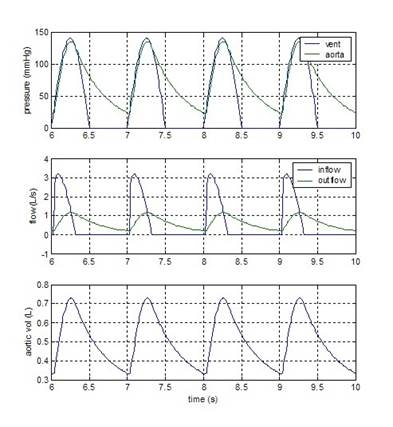Figure 6 Pressures, flows and volumes for a compliant aorta

These effects due to the compliance of the aorta are known as WindKessel effects.  They can affect pressures and flow rates in the arterial system as the compliance of the arteries change due to either physiological (e.g., contraction of the arterial smooth muscles) or pathological (e.g., atherosclerosis “hardening of the arteries”) processes.

In this exercise, we will examine the role of the stiffness of an artery and the resistance of the distal (= downstream) vessel in modulating pulsatile flow through the vessel.

#### Model of flow in an artery

Suppose we want to know what pressures and flow rates will be seen in an artery (e.g., to study exercise physiology, the physiology of shock, the pathogenesis of atherosclerosis, etc.).  The physics of the situation is, in simplified form, rather easy.

1)    We can approximate flow through a pipe as the pressure head divided by the resistance.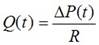(5)

where         Q(t)   = flow at time t    (L/s)

)P(t) = pressure head (Pupstream - Pdownstream)   (mmHg)

The resistance is usually assumed to be approximately constant.  This formula works reasonably well for rigid pipes with reasonably steady flows.

2)    But an artery is not rigid.  Both the pressure and the volume in the artery "pulse" over time,pushing against the elastic walls of the vessel.  If pressure and volume change slowly enough, they can be approximated by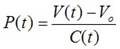(6)

where         V(t)            = volume of blood in the vessel at time t    (L) V0            = volume of vessel when P = 0

(dead, null, or unstressed volume)    (L) C(t)       = compliance of artery   (L/mmHg)

Compliance is usually assumed constant both through time and versus pressure, neither of which is strictly true.

In order to deal with the fact that pressures and volumes can (and do) change through the course of the cardiac cycle, we'll set up a series of equations, using a common trick in biological simulations, called a balance equation - in this case, we'll do a mass balance on the artery

)Volume / )t  = InFlow - OutFlow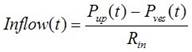(7a)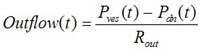(7b)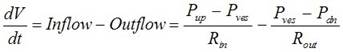(7c)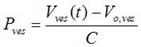(8)

Note that in this description, Rin might vary with time because it might include the resistance of the aortic backflow check valve.

1)    Of the quantities that vary in time (i.e., variables - as opposed to those that are fixed (calledparameters)), which quantities do we need to know to give a complete description of the state of the systems?

2)    It would appear that the solution to the system of equations (Eq. 7c and 8) involves integration ofsome derivatives (i.e., we seek two functions (Vart(t) and Part(t)) that make Eqs. 7c and 8 true.  How is this problem different from those you learned about in calculus classes?

#### Simulations

We’ll hook our model artery to a ventricle (with an aortic valve) that provides a series of pressure pulses (unlike a real ventricle, the pressure here does not depend on the amount of blood pumped), and examine

the pressures, flows, and volumes in the arteries.  We’ll carry out the actual simulations in a modelling package called “SIMULINK” that sits on top of MATLAB and talks to MATLAB.  SIMULINK is a “box and arrow” modelling program - that is one simulates mathematical processes by drawing diagrams of the processes involved, and lets SIMULINK do the work of dealing with the differential equations.  This is a common way of exploring models in biology and it’s worth seeing it, although we won’t dig very deeply into it.  The box and arrow diagram for our model is given in Figs. 7 and 8.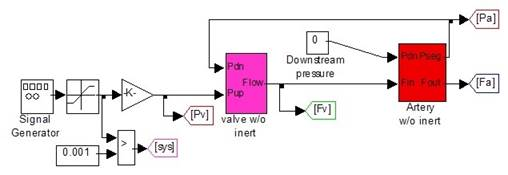Figure 7.  Overall system diagram for WindKessel simulation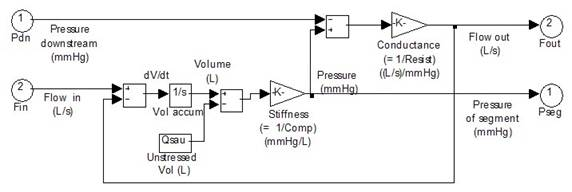Figure 8.  Subsystem diagram for artery (WindKessel model)

In Fig. 7, the left hand “blocks” represent the ventricular pressure generator and we needn’t worry about their details.  The block labeled “valve w/o inert” is a subsystem (SIMULINK’s jargon for a submodel) that represents the aortic valve and prevents backflow from the aorta to the ventricle.  It uses the ventricular pressure and the aortic pressure to calculate the flow from the ventricle to the aorta.  Again, we don’t need to worry about the details.  The block that is labeled “Artery w/o inert” is our artery.  Here we do need some details.

Double clicking on the artery block yields Fig. 8.  This is the subsystem that represents the artery and codes Eq. 7-8.  The easiest way to see this is to start just to the left of the block labeled “vol accum.”  The input line there is labeled dV/dt.  Eq. 7c tells us that dV/dt should be inflow minus outflow, and in fact, the block just to the left of dV/dt sums two inputs labeled ‘flow in’ and ‘flow out’.  ‘Flow in’ is an input (it is calculated by the valve block).  ‘Flow out’ is calculated elsewhere in the subsystem; we’ll come back to that.  The ‘vol accum’ block is an integrator, i.e., it integrates the derivative (dV/dt) to give, as an output, the volume of the artery at any given time.  Having obtained that volume, we can subtract the unstressed volume and multiply by the stiffness  to get the pressure in the artery (Eq. 8).  SIMULINK multiplies by a constant via a ‘gain’ block (the triangle shaped block labeled ‘stiffness’).  Remember stiffness is

1/compliance, so multiplying by the stiffness is the same as dividing by the compliance.  Now we have the pressure in the artery.  We can subtract the downstream pressure (an input) and multiply by the conductance (= divide by the resistance) to get the outflow rate (Eq. 7b).  Recall that this rate is fed back to the block that calculates dV/dt.  The difference between the upstream (ventricular) pressure and the aortic pressure is calculated and divided by the aortic valve resistance in the valve block (Fig. 6) to provide the inflow as an input (Fig. 8).

The values of the stiffness and the conductance are entered into the model by double clicking on the appropriate gain blocks (Fig. 8).  In this case the names of variables (‘Swa’ for stiffness, ‘Cwa’ for conductance) have already been entered, and all you need to do is set the values of the variables.  They can be set by running the program called WindKpar.m (for Wind Kessel Parameters) in MATLAB.

Outputs from the model are saved in the MATLAB workspace by the output section of the model (Fig. 9).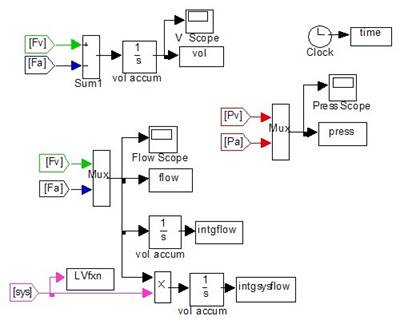Figure 9.  Output section of the model.

The output section takes values generated by the simulation (in the colored ‘goto’ and ‘from’ flags) and stores them in the MATLAB workspace.  It also graphs some of them in quick plots SIMULINK calls scopes.  At each time step, it takes the ventricular and arterial pressures and dumps them into a variable called “press”, each time step becomes a row and each pressure a column in the output variable (the times at each time step are stored in a variable cleverly called “time”.  Likewise the flows from the ventricle into the artery (‘Fv’) and downstream from the artery (‘Fa’) are stored in a variable called “flow”.  Finally, the outflow (‘Fa’) is subtracted from the inflow (‘Fv’) to estimate dV/dt and that derivative integrated to estimate the volume of the artery at each time (stored in “vol”).

#### Running a simulation -

1)  Set your MATLAB current directory to the folder that contains the files WindKpar.m and

SimpWind.mdl.  In the MATLAB command window, type WindKpar

to run the program that sets parameters for the simulation.

2)  Adjust the values of ‘Swa’ and ‘Cwa’ if needed, see below.

3)  Open SimpWind.mdl if you have not already done so.

4)  Click on the right pointing arrow in the toolbar at the top of the window to start the simulation.

5)  Double click on the “scopes” (Fig. 9) to examine the outcomes.

6)  At the MATLAB command window, type

Windksimplot

to get a plot of the key variables.  Copy and save this plot as needs be.  Examine the pressures, flow, and arterial volume.

7)  You can save the values from the simulation if you need to do so.  The values are in a series ofvariables in MATLAB’s workspace.

save filename.txt time press flow vol intgflow Lvfxn intgsysflow -ascii -tabs should save most of the important information.

Simulations

1)  You should run simulations as above for several different values of stiffness (‘Swa’) to see howchanging the stiffness (= 1/compliance) affects pressures, flows, and volumes.  Make sure you can explain mechanistically the differences you see.

2)  Run the program ‘windksimrun.m’ to explore the effects of changing arterial compliance andconductance over a range of values.  The program runs the SIMULINK model ‘simpwind.mdl’ for a whole series of values of compliances and resistances and plots the results.  Can you explain the results?  You can run ‘simpwind.mdl’ for any combinations of compliance and resistance that you find confusing or important.

## Assignment Description

WindKessel Model

Questions

1)      Explain the Windkessel effect using Arterial Flow, Pressure and Volume.

Trace the parameter’s pattern with time in the figures generated by Matlab( SimpWind.mdl). Compare it with patterns of a lead pipe and a water baloon. Justify the differences.

2)      Define Resistance and Compliance. What variables are affected by the changes in both Resistance and Compliance? Explain how these combination of changes have an effect on the arterial parameters and Why?

Use results from windksimrun. Observe and state the changes in trends and use the theory discussed on reference material to justify these changes. Refer back to Wind Kes Indiv Results.

3)     What physiological conditions cause such a change in resistance and compliance?

4)     Of the quantities that vary in time (i.e., variables - as opposed to those that are fixed (called

parameters), which quantities do we need to know to give a complete description of the state of

the systems? Use  results from SimpWind.mdl

## Assignment Description

Resist = 5    Comp =0.1                      Comp = 0.3                 Comp = 1                    Comp = 3           Comp = 10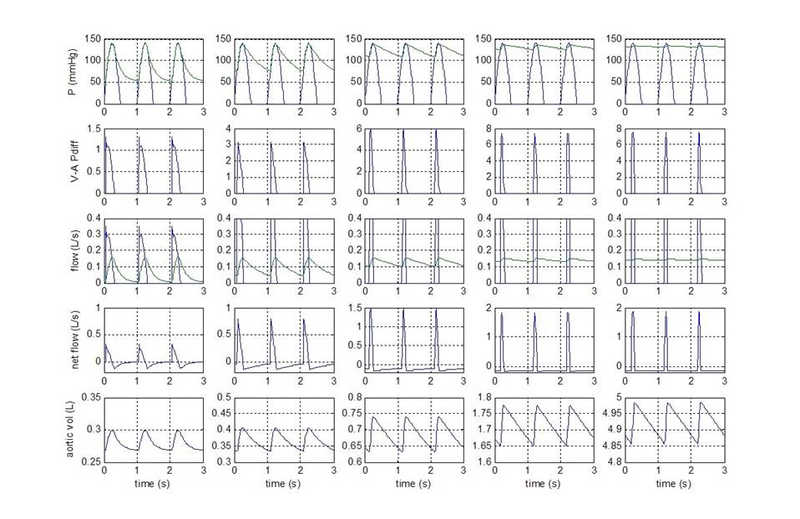Resist = 1    Comp =0.1                      Comp = 0.3                 Comp = 1                                Comp = 3    Comp = 10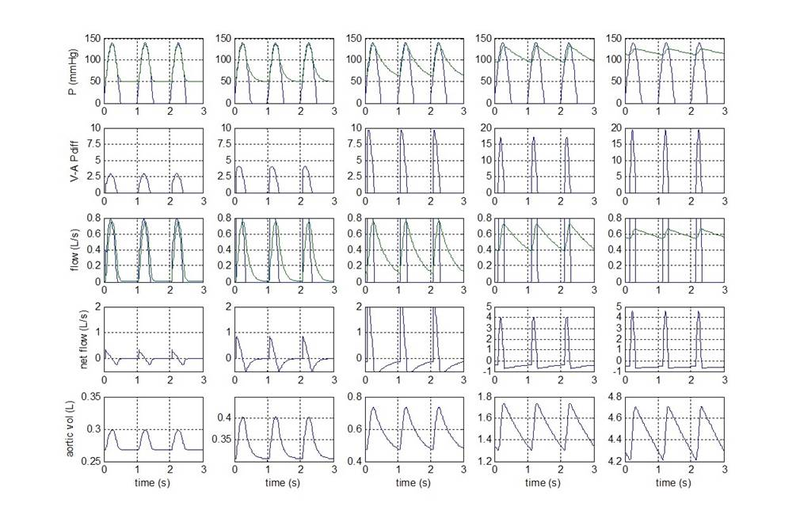Resist = 0.2    Comp =0.1                   Comp = 0.3                 Comp = 1                                Comp = 3    Comp = 10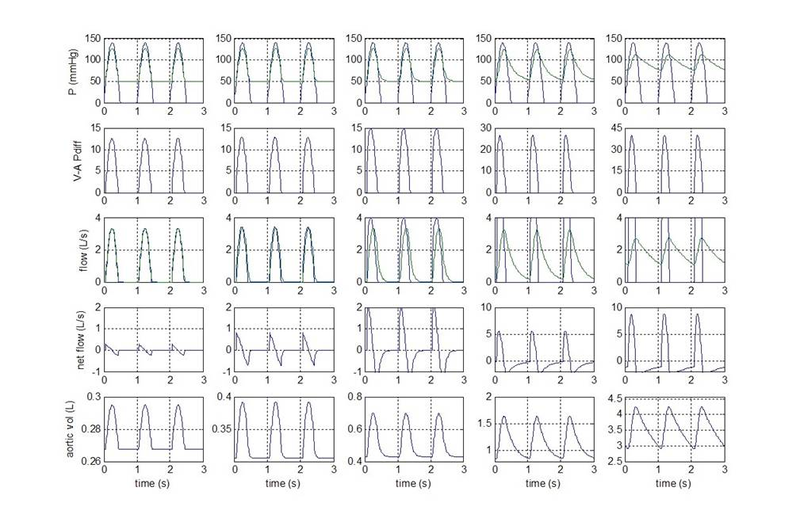## Assignment Code

``````
HR   = 83.2;		%bpm			Heart rate
fsys = 0.4;			%				fraction of heart beat in systole
Slvd = 67/1.333;	%mmHg/L			LV diastolic stiffness
Slvs = 2500/1.333;	%mmHg/L			LV systolic stiffness

PAtc = 7.2;			%mmHg			constant atrial pressure
RAV  = 5.0/1.333;	%mmHg/(L/s)		AV resistance
Cav  = 1/RAV;		%(L/s)/mmHg		AV conductance
Pvec = 3;			%mmHg			constant venous pressure

Rlv  = 5.0/1.333;	%mmHg/(L/s)		LV - aortic resistance
Clv  = 1/Rlv;		%(L/s)/mmHg		Aortic conductance
Llv  = 0.5/1.333;	%mmHg s2/L		inertance in left ventricle		0.5/1.333;
Plv  = 1/Llv;		%				permitance in left ventricle

RLa  = 5/1.333;		%mmHg/(L/s)		resistance in LAtrium		5/1.333;
CLa  = 1/RLa;		%(L/s)/mmHg		conductance in LAtrium
CpLa = 0.01176*1.333;	%L/mmHg		capacitance in LAtrium	0.01176*1.333;
SLa  = 1/CpLa;		%mmHg/L			stiffness in LAtrium
QLau = 0.050;		%L				unstressed volume of LAtrium
LLa  = 0.5/1.333;	%mmHg s2/L		inertance in LAtrium		0.5/1.333;
PLa  = 1/LLa;		%				permitance in LAtrium

RAo  = 50/1.333;	%mmHg/(L/s)		resistance in aorta		50/1.333;
Cao  = 1/RAo;		%(L/s)/mmHg		conductance in aorta
Cpao = 1.5e-4*1.333;	%L/mmHg		capacitance in aorta	1.5e-4*1.333;
Sao  = 1/Cpao;		%mmHg/L			stiffness in aorta
Qaou = 0.085;		%L				unstressed volume of aorta
Lao  = 0.5/1.333;	%mmHg s2/L		inertance in aorta		0.5/1.333;
Pao  = 1/Lao;		%				permitance in aorta

RSA  = 80/1.333;	%mmHg/(L/s)		resistance in large arteries
Csa  = 1/RSA;		%(L/s)/mmHg		conductance in large arteries
Cpsa = 3e-4*1.333;	%L/mmHg			capacitance in slarge arteries
Ssa  = 1/Cpsa;		%mmHg/L			stiffness in large arteries
Qsau = 0.250;		%L				unstressed volume of large arteries
Lsa  = 1.35/1.333;	%mmHg s2/L		inertance in large arteries		1.35/1.333;
Psa  = 1/Lsa;		%				permitance in large arteries

RCA  = 1150;		%mmHg/(L/s)		resistance in small arteries
Cca  = 1/RCA;		%(L/s)/mmHg		conductance in small arteries
Cpca = 2.2e-3*1.333;	%L/mmHg		capacitance in small arteries	2.2e-3*1.333;
Sca  = 1/Cpca;		%mmHg/L			stiffness in small arteries
Qcau = 0.810;		%L				unstressed volume of small arteries
Lca  = 5/1.333;		%mmHg s2/L		inertance in small arteries		5/1.333;
Pca  = 1/Lca;		%				permitance in small arteries

Rv   = 100/1.333;	%mmHg/(L/s)		resistance in veins		100/1.333;
Cv   = 1/Rv;		%(L/s)/mmHg		conductance in veins
Cpv  = 0.045*1.333;	%L/mmHg			capacitance in veins	0.037*1.333;
Sv   = 1/Cpv;		%mmHg/L			stiffness in veins
Qvu  = 2.860;		%L				unstressed volume of veins
Lv   = 10/1.333;	%mmHg s2/L		inertance in veins		10/1.333;
Pv   = 1/Lv;		%				permitance in veins

QLa0 = 0.105;
Qlv0 = 0.120;
Qao0 = 0.100;
Qsa0 = 0.281;
Qca0 = 1.010;
Qv0  = 3.66;

Cwa  = Cca*10;
Swa  = 1/(Cpao+Cpsa+Cpca);

``````

## Assignment Code

``````
cur = find(time >= 6.0);
figure
subplot(3,1,1)
plot(time(cur),press(cur,:),'linewidth',2)
ylabel('pressure (mmHg)')
legend('vent','aorta')
grid
subplot(3,1,2)
plot(time(cur),flow(cur,:),'linewidth',2)
ylabel('flow (L/s)')
legend('inflow','outflow')
grid
subplot(3,1,3)
plot(time(cur),vol(cur),'linewidth',2)
ylabel('aortic vol (L)')
xlabel('time (s)')
grid

``````

## Assignment Code

``````
cur = find(time >= (max(time)-6.0));
figure
subplot(5,1,1)
plot(time(cur),press(cur,:),'linewidth',2)
ylabel('P (mmHg)')
legend('vent','aorta')
grid
subplot(5,1,2)
plot(time(cur),press(cur,1)-press(cur,2),'linewidth',2)
ylabel('V-A Pdiff')
grid

subplot(5,1,3)
plot(time(cur),flow(cur,:),'linewidth',2)
ylabel('flow (L/s)')
legend('inflow','outflow')
grid
subplot(5,1,4)
plot(time(cur),flow(cur,1)-flow(cur,2),'linewidth',2)
ylabel('net flow (L/s)')
grid

subplot(5,1,5)
plot(time(cur),vol(cur),'linewidth',2)
ylabel('aortic vol (L)')
xlabel('time (s)')
grid

``````

## Assignment Code

``````

windKpar

Cbase  = Cwa;			%(L/s)/mmHg		peripheral conductance
Sbase  = Swa;			%mmHg/L			stiffness in arteries

% stiffvec = [0.1 0.2 0.5 1 2 5 10]';
stiffvec = logspace(-1,1,41)';
nstiff = length(stiffvec);
condvec = [0.1 0.2 0.5 1 2 5 10]';
ncond  = length(condvec);
stiffsource = stiffvec * ones(size(condvec'));
condsource  = ones(size(stiffvec)) * condvec';
Svec = stiffsource(:);
Cvec = condsource(:);
parammat = [Cvec Svec];

[npset,ncol] = size(parammat);
outmat = [];

for ploc = 1:npset,
ploc
params = parammat(ploc,:);
Cwa	= Cbase * params(1);
Swa	= Sbase * params(2);

[t,x,y] = sim('simpwind', [0 60]);
[nrow,ncol] = size(intgflow);
ntime = length(t);
tout  = t((ntime-nrow+1):ntime);

tED = tout(find(diff(LVfxn(2:nrow,1)) > 0)-1);
ntED = length(tED);
tED5 = tED((ntED-4):ntED);

delt5 = tED5(5)- tED5(1);
cFLWi = interp1(tout,intgflow,tED5(1));
cFLWf = interp1(tout,intgflow,tED5(5));
mnFLW = (cFLWf - cFLWi)/delt5;
sFLWi = interp1(tout,intgsysflow,tED5(1));
sFLWf = interp1(tout,intgsysflow,tED5(5));
fracfsys = (sFLWf - sFLWi) ./ (cFLWf - cFLWi);

latet = find(tout > tED5(1));
maxP  = max(press(latet,:));
minP  = min(press(latet,:));
maxFl  = max(flow(latet,:));
minFl  = min(flow(latet,:));
maxVl  = max(vol(latet,:));
minVl  = min(vol(latet,:));

outmat = [outmat; [params mnFLW maxFl minFl fracfsys maxP minP maxVl minVl]];
end;   % for ploc
avflws = outmat(:,4);
mxflws = outmat(:,6);
mnflws = outmat(:,8);
frflws = outmat(:,10);
mxprs  = outmat(:,12);
mnprs  = outmat(:,14);
mxvol  = outmat(:,15);
mnvol  = outmat(:,16);
rtvol  = (mxvol - mnvol) ./ mnvol;
% plot results - x axis = compliance,  legend = resistance
figure
subplot(2,2,1);   semilogx(1 ./stiffvec, reshape(mxflws,nstiff,ncond));
grid
xlabel('compliance (* baseline)');  ylabel('max flow')
subplot(2,2,2);   semilogx(1 ./stiffvec, reshape(mnflws,nstiff,ncond));
grid
xlabel('compliance (* baseline)');  ylabel('min flow')
legend('10','5','2','1','0.5','0.2','0.1')
subplot(2,2,3);   semilogx(1 ./stiffvec, reshape(avflws,nstiff,ncond));
grid
xlabel('compliance (* baseline)');  ylabel('mean flow')
subplot(2,2,4);   semilogx(1 ./stiffvec, reshape(frflws,nstiff,ncond));
grid
xlabel('compliance (* baseline)');  ylabel('frac flow in systole')

figure
subplot(2,2,1);   semilogx(1 ./stiffvec, reshape(mxprs,nstiff,ncond));
grid
xlabel('compliance (* baseline)');  ylabel('max pressure')
subplot(2,2,2);   semilogx(1 ./stiffvec, reshape(mnprs,nstiff,ncond));
grid
xlabel('compliance (* baseline)');  ylabel('min pressure')
subplot(2,2,3);   loglog(1 ./stiffvec, reshape(mnvol,nstiff,ncond));
hold on
loglog(1 ./stiffvec, reshape(mxvol,nstiff,ncond), '--');
grid
xlabel('compliance (* baseline)');  ylabel('art vol (max and min)')
subplot(2,2,4);   semilogx(1 ./stiffvec, reshape(rtvol,nstiff,ncond));
grid
xlabel('compliance (* baseline)');  ylabel('delta vol / min vol')

``````

## Assignment Code

``````

windKpar

Cbase  = Cwa;			%(L/s)/mmHg		peripheral conductance
Sbase  = Swa;			%mmHg/L			stiffness in arteries

% stiffvec = [0.1 0.2 0.5 1 2 5 10]';
stiffvec = [0.1 sqrt(10)/10 1 sqrt(10) 10]';
stiffvec = flipud(stiffvec);
nstiff = length(stiffvec);
condvec = [0.2 1 5]';
ncond  = length(condvec);
timescx = 3;

tic
for Cndct = 1:ncond,
Cwa	= Cbase * condvec(Cndct);
figure

for Stfct = 1:nstiff,
Swa	= Sbase * stiffvec(Stfct);

[t,x,y] = sim('simpwind', [0 60]);

cur = find(time >= (max(time)-timescx));
subplot(5,nstiff,((1-1)*nstiff+Stfct))
plot(time(cur)-(max(time)-timescx),press(cur,:))
if (Stfct == 1),
ylabel('P (mmHg)'); end;
%         legend('vent','aorta')
axlim = axis;  axis([0 timescx axlim(3:4)]);
grid
subplot(5,nstiff,((2-1)*nstiff+Stfct))
plot(time(cur)-(max(time)-timescx),max(0,[press(cur,1)-press(cur,2)]))
if (Stfct == 1),
ylabel('V-A Pdiff'); end;
axlim = axis;  axis([0 timescx axlim(3:4)]);
grid
hold on

subplot(5,nstiff,((3-1)*nstiff+Stfct))
plot(time(cur)-(max(time)-timescx),max(0,[flow(cur,:)]))
if (Stfct == 1),
ylabel('flow (L/s)'); end;
%         legend('inflow','outflow')
axlim = axis;  axis([0 timescx axlim(3:4)]);
grid
subplot(5,nstiff,((4-1)*nstiff+Stfct))
plot(time(cur)-(max(time)-timescx),flow(cur,1)-flow(cur,2))
if (Stfct == 1),
ylabel('net flow (L/s)'); end;
axlim = axis;  axis([0 timescx axlim(3:4)]);
grid

subplot(5,nstiff,((5-1)*nstiff+Stfct))
plot(time(cur)-(max(time)-timescx),vol(cur))
if (Stfct == 1),
ylabel('aortic vol (L)'); end;
xlabel('time (s)')
axlim = axis;  axis([0 timescx axlim(3:4)]);
grid
end;  % for Stfct
end;   % for Cndct
toc

``````

Is it free to get my assignment evaluated?

Yes. No hidden fees. You pay for the solution only, and all the explanations about how to run it are included in the price. It takes up to 24 hours to get a quote from an expert. In some cases, we can help you faster if an expert is available, but you should always order in advance to avoid the risks. You can place a new order here.

How much does it cost?

The cost depends on many factors: how far away the deadline is, how hard/big the task is, if it is code only or a report, etc. We try to give rough estimates here, but it is just for orientation (in USD):

 Regular homework \$20 - \$150 Advanced homework \$100 - \$300 Group project or a report \$200 - \$500 Mid-term or final project \$200 - \$800 Live exam help \$100 - \$300 Full thesis \$1000 - \$3000

How do I pay?

Credit card or PayPal. You don't need to create/have a Payal account in order to pay by a credit card. Paypal offers you "buyer's protection" in case of any issues.

Why do I need to pay in advance?

We have no way to request money after we send you the solution. PayPal works as a middleman, which protects you in case of any disputes, so you should feel safe paying using PayPal.

Do you do essays?

No, unless it is a data analysis essay or report. This is because essays are very personal and it is easy to see when they are written by another person. This is not the case with math and programming.

Why there are no discounts?

It is because we don't want to lie - in such services no discount can be set in advance because we set the price knowing that there is a discount. For example, if we wanted to ask for \$100, we could tell that the price is \$200 and because you are special, we can do a 50% discount. It is the way all scam websites operate. We set honest prices instead, so there is no need for fake discounts.

Do you do live tutoring?

No, it is simply not how we operate. How often do you meet a great programmer who is also a great speaker? Rarely. It is why we encourage our experts to write down explanations instead of having a live call. It is often enough to get you started - analyzing and running the solutions is a big part of learning.

What happens if I am not satisfied with the solution?

Another expert will review the task, and if your claim is reasonable - we refund the payment and often block the freelancer from our platform. Because we are so harsh with our experts - the ones working with us are very trustworthy to deliver high-quality assignment solutions on time.

Customer Feedback

"Thanks for explanations after the assignment was already completed... Emily is such a nice tutor! "

Order #13073